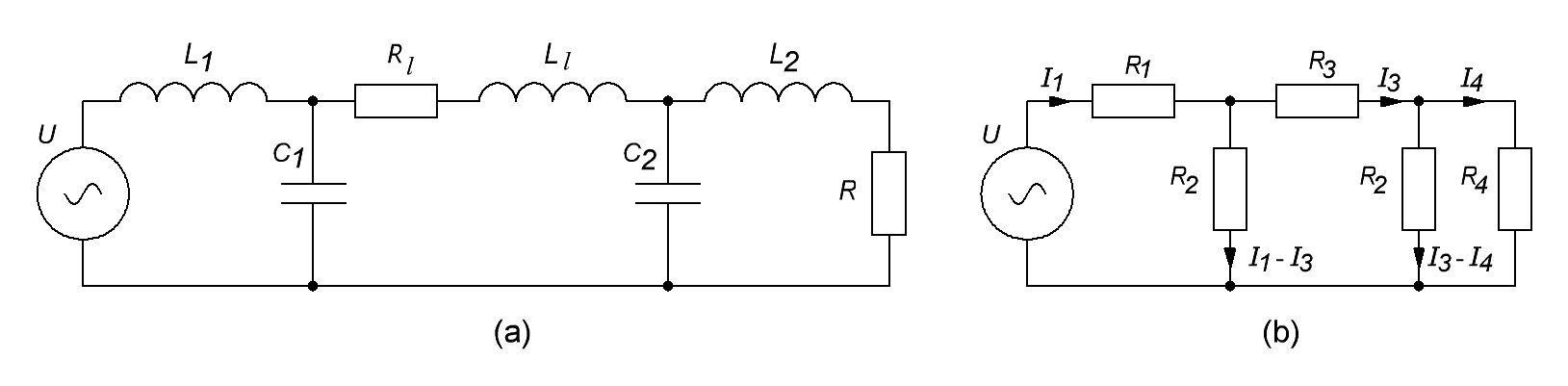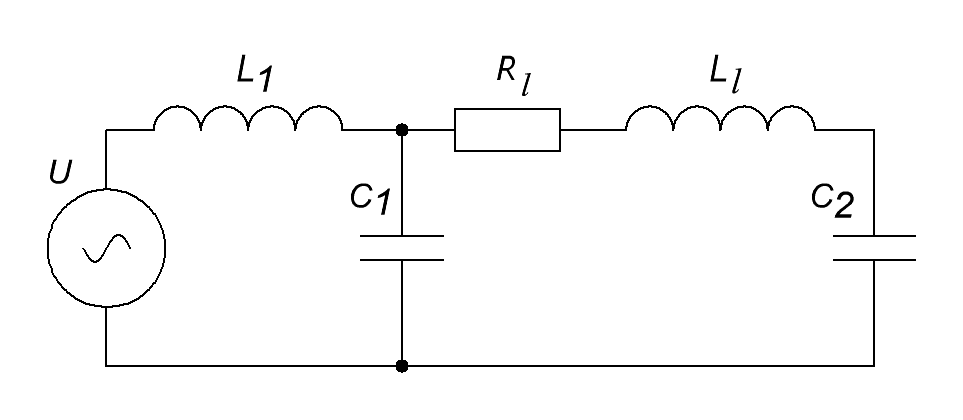Research website of Vyacheslav Gorchilin
2020-12-30
3. Conduction current transfer characteristic
According to the conditions of the problem, the line length is much less than the wavelength, and therefore the circuit parameters are considered lumped. Since the scheme of the transmitter, receiver and power lines can be reduced to an equivalent one, as it is done in Figure 6a.Fig.6. Equivalent ROES scheme (a) and calculation scheme (b)
In this diagram, $$L_1$$ is the inductance of the secondary winding of the spin transformer TS1 , which is connected to the communication line. The line itself is presented here in the form of a chain: $$C_1, C_2, R_l, L_l$$, where $$C_1 + C_2 = C_l$$ is the capacity of the transmission line, $$R_l$$ is its active resistance, $$L_l$$ - its inductance. $$L_2$$ - represents the inductance of the receiving winding of the broadband transformer TS2 , and $$R$$ is its active load.
It is more convenient to represent the scheme for the Kirchhoff calculation as shown in Figure 6b. In this circuit, the following replacements are introduced: $$R_1 = i \omega L_1$$, $$R_2 = 1 / (i \omega C_1) = 2 / (i \omega C)$$, $$R_3 = i \omega L_l + R_l$$, $$R_4 = i \omega L_2 + R$$. From here, you can compose the following system of equations: $\begin{equation*} \begin {cases} U = I_1 R_1 + (I_1 - I_3) R_2 \\ 0 = I_3 R_3 + (I_3 - I_4) R_2 - (I_1 - I_3) R_2 \\ 0 = I_4 R_4 - (I_3 - I_4) R_2 \end {cases} \end{equation*} \qquad (3.1)$ By transformations, we can find all the currents in this circuit: $\begin {equation*} \begin {cases} I_1 = U {A \over B} \\ I_3 = I_1 {R_2 (R_2 + R_4) \over A} \\ I_4 = I_1 {R_2 ^ 2 \over B} \end {cases} \end {equation*} \qquad (3.2)$ Where: $\begin {equation*} \begin {cases} A = R_3 (R_2 + R_4) + R_2 (2 R_4 + R_2) \\ B = A (R_1 + R_2) - R_2 ^ 2 (R_2 + R_4) \end {cases} \end {equation*} \qquad (3.3)$ Then the transfer characteristic will be found as the ratio of the output and input power: ${P_2 \over P_1} = {R , R_2 ^ 4 \over A , B} \qquad (3.4)$ Although this approach rather accurately reflects the characteristics of the circuit, it is rather cumbersome and difficult to analyze. Further, with some assumptions, we will simplify the circuit a little and find its resonant frequency.
4. Resonant frequency
By simulating the circuit shown in Figure 6a in MicroCap, it was possible to find out that the influence of $$L_2$$ is the winding of the broadband transformer TS2 and the load $$R$$, practically does not affect the value of the resonant frequency (within reasonable limits). Therefore, to find it, we can simplify this scheme to the one shown in Figure 7.Fig.7. Simplified line diagram for calculating the resonant frequency
Let's introduce a coefficient that will show us the ratio of the inductances of the winding of the transformer TS1 and the line, $k = {L_1 \over L_l} \qquad (4.1)$ and then, by transforming the formulas from Section 3, we find the resonant frequency of the circuit: $\omega = \sqrt {{1 + 2k - \sqrt {4k ^ 2 + 1} \over k L_l C_l}} \qquad (4.2)$ Here: $$\omega = 2 \pi f$$. This expression can be simplified if we assume that $$k$$ is much greater than one, which corresponds to real conditions. Then the resonant frequency will be approximately as follows: $\omega \approx {1 \over \sqrt {k L_l C_l}} = {1 \over \sqrt {L_1 C_l}} \qquad (4.3)$ From formula (4.3) it follows that the main contribution to the resonant frequency is made by the inductance of the output winding of the spin transformer TS1 and the capacity of the transmission line. The same frequency will be the working one for the master sinusoidal generator Ug (Fig. 2a).
4.1. Another option for calculating the displacement power
From formula (4.3) it follows that the main contribution to the resonant frequency is made by the inductance of the transformer TS1 and the capacity of the transmission line. If we substitute formula (4.3) into (1.29), then we get a fairly unique ratio for calculating the maximum displacement power: $P_D \approx {U ^ 2 \over Z \sqrt {k}} \qquad (4.4)$ where $$Z$$ is the wave impedance of the transmission line. Here we immediately take the root-mean-square (effective) voltage value in the line $$U$$.
Also, the following form of writing the same formula may be interesting: $P_D \approx U ^ 2 \sqrt {C_p l \over L_1} \qquad (4.5)$ where $$C_p$$ is the specific capacity of the line (F/m), $$l$$ is its length (m).
For a line in the form of a coaxial cable, formula (4.5) seems to us the most convenient for quickly calculating its displacement power, especially for long lines, when the contribution of this power is the most significant. Then, to find it, we just need to know the inductance of the output winding and the output voltage of the transformer TS1 (Fig. 2), the specific nameplate capacity and the length of the cable. It should be recalled that in this case the entire system is in resonance, the frequency of which is calculated by formula (4.2) or (4.3). Also, we assume that the length of the transmission line is much less than its wavelength.

Materials used
1. Kotelnikov V.A., Nikolaev A.M. Fundamentals of radio engineering. Chapter 9. Linked paths.